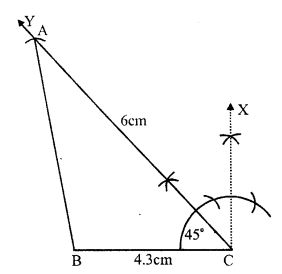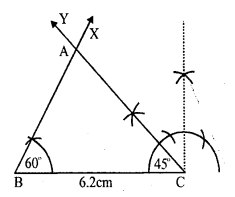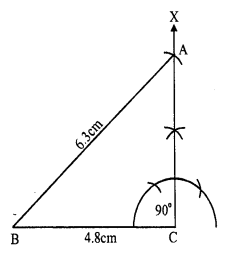# RS Aggarwal Class 7 Solutions Chapter 17 Constructions Ex 17B

In this chapter, we provide RS Aggarwal Solutions for Class 7 Chapter 17 Constructions Ex 17B for English medium students, Which will very helpful for every student in their exams. Students can download the latest RS Aggarwal Solutions for Class 7 Chapter 17 Constructions Ex 17B Maths pdf, free RS Aggarwal Solutions Class 7 Chapter 17 Constructions Ex 17B Maths book pdf download. Now you will get step by step solution to each question.

### RS Aggarwal Solutions for Class 7 Chapter 17 Constructions Ex 17B Download PDF

Question 1.
Solution:
Steps of construction :
(i) Draw a line segment BC = 3.6cm(ii) At B, draw an arc of the radius 5cm.
(iii) At C, draw another arc of the radius 5.4cm which intersects the first arc at A
(iv) Join AB and AC
(v) With centre B and C and radius more than half of BC, draw arcs intersecting each other at L and M.
(vi) Join LM which intersects BC at Q and produce it to P.
Then PQ is perpendicular bisector of side BC.

Question 2.
Solution:
Steps of construction :
(i) Draw a line segment QR = 6cm
(ii) With centre Q and radius 4.4 cm draw an arc.
(iii) With centre R and radius 5.3 cm, draw another arc intersecting the first arc at P.
(iv) Join PQ and PR.(v) With centre P and a suitable radius, draw an arc meeting PR at E and PQ at F.
(vi) With centres E and F, with same radius, draw two arcs intersecting each other at G.
(vii) Join PG and produce it to meet QR at S. Then PS is the bisector of ∠P.

Question 3.
Solution:
Steps of construction :
(i) Draw a line segment BC = 6.2 cm.
(ii) With centres B and C radius 6.2 cm, draw arcs intersecting each other at A.
(iii) Join AB and AC.∆ABC is the required equilateral triangle.
On measuring, each angle is equal to 60°.

Question 4.
Solution:
Steps of construction :
(i) Draw a line segment BC = 5.3 cm.
(ii) With centre B and C, and radius 4.8 cm, draw arcs intersecting each other at A
(iii) Join AB and AC, Then ∆ABC is the required triangle
(v) Then with centre L and M, draw two arcs intersecting eachother at E.
(vi) Join AE intersecting BC at D. Then AD is perpendicular to BC.

On measuring ∠B and ∠C, each is equal to 55°.

Question 5.
Solution:
Steps of construction :
(i) Draw a line segment AB = 3.8cm.
(ii) At A draw a ray AX making an angle of 60°.(iii) Cut off AC = 5 cm from AX
(iv) Join CB.
Then ∆ABC is the required triangle.

Question 6.
Solution:
Steps of construction :
(i) Draw a line segment BC = 4.3 cm.
(ii) At C, draw a ray CY making an angle equal to 45°.(iii) With centre C, and radius 6cm, draw an arc intersecting CY at A.
(iv) Join AB.
Then ∆ABC is the required triangle.

Question 7.
Solution:
(i) Draw a line segment AB = 5.2cm.
(ii) At A, draw a ray AX making an angle equal to 120°.(iii) From AX cut off AC = 5.2cm.
(iv) Join BC.
Then ∆ABC is the required triangle.
(v) With centre A and some suitable radius draw an arc intersecting BC at L and M.
(vi) With centres L and M, draw two arcs intersecting each other at E.
(vii) Join AE intersecting BC at D Then AD is the perpendicular to BC.

Question 8.
Solution:
Steps of construction :
(i) Draw a line segment BC = 6.2 cm.
(ii) At B draw a ray BX making an angle of 60°.(iii) At C draw another ray CY making an angle of 45° which intersect the ray BX at A. .
Then ∆ABC is the required triangle.

Question 9.
Solution:
Steps of construction :
(i) Draw a line segment BC = 5.8 cm.
(ii) At B draw a ray BX making an angle of 30°(iii) At C draw another ray CY making an angle of 30° intersecting the BX at A
Then ∆ABC is the required triangle.
On measuring AB and AC, AB = 3.5cm and AC = 3.5cm.
AB = AC
∆ABC is an isosceles triangle.

Question 10.
Solution:
Steps of construction :
In ∆ABC, ∠A = 45° and ∠C = 75°
But ∠A + ∠B + ∠C = 180°⇒ 45° + ZB + 75° = 180°
⇒ ∠B = 180° – 45° – 75°
⇒ ∠B = 180° – 120° = 60°
(i) Draw a line segment AB = 7cm.
(ii) At A, draw a ray AX making an angle of 45°.
(iii) At B, draw another ray BY making an angle of 60° which intersects AX at C
Then ∆ABC is the required triangle.

Question 11.
Solution:
Steps of construction :
(i) Draw a line segment BC = 4.8 cm
(ii) At C, draw a ray CX making an angle of 90°.(iii) With centre B, an radius 6.3cm draw an arc intersecting CX at A.
(iv) Join AB.
Then ∆ABC is the required triangle.

Question 12.
Solution:
Steps of construction :
(i) Draw a line segment BC = 3.5cm
(ii) At B, draw a ray BX making an angle of 90°.(iii) With centre C and radius 6cm draw an arc intersecting BX at A
(iv) Join AC.
Then ∆ABC is the required triangle.

Question 13.
Solution:
One acute angle = 30°, then
second acute angle will be = 90° – 30° = 60° (Sum of acute angles = 90°)
Steps of construction :
(i) Draw a line segment BC = 6cm.
(ii) At B draw a ray BX making an angle of 30°.
(iii) At C draw another ray CY making an angle of 60° which intersects BX at AThen ∆ABC is the required triangle.

All Chapter RS Aggarwal Solutions For Class 7 Maths

—————————————————————————–

All Subject NCERT Exemplar Problems Solutions For Class 7

All Subject NCERT Solutions For Class 7

*************************************************

I think you got complete solutions for this chapter. If You have any queries regarding this chapter, please comment on the below section our subject teacher will answer you. We tried our best to give complete solutions so you got good marks in your exam.

If these solutions have helped you, you can also share rsaggarwalsolutions.in to your friends.